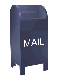## The Wavelet Packet Transform

View the Doxygen Generated Documentation

### Introduction

As the title of the page sugests, this web page describes the wavelet packet transform. The wavelet packet transform has a number of applications. One of these involves the calculation of the "best basis", which is a minimal representation of the data relative to a particular cost function. The "best basis" is used in applications that include noise reduction and in data compression.

This web page also publishes C++ code that implements the wavelet packet transform, the best basis calculation and the inverse transform from the best basis set.

In my wanderings through the literature on wavelets, I have found the wavelet packet transform one of the most difficult topics to understand. Frequently the wavelet packet transform is described in terms like this:

In this chapter we consider the discrete wavelet packet transform (DWPT), which can be regarded as any one of a collection of orthonormal transforms, each of which can be readily computed using a very simple modification of the pyramid algorithm for the DWT [Discrete Wavelet Transform]

...

As in Chapter 4, let W = WX represent the wavelet coefficients obtained by transforming X using the N x N orthonormal DWT matrix W (for convenience, we assume that N = 2j for some integer J).

The quotes above are from sections 6.0 and 6.1 of Wavelet Methods for Time Series Analysis by Percival and Walden, Cambridge University Press, 2000

Wavelet Methods is an good book that provides a view of wavelet mathematics through the glass of linear algebra. However, while linear algebra provides a compact way to express wavelet mathematics, wavelet algorithms are not implemented using linear algebra techniques.

The description of the wavelet packet transform presented here relies heavily Chapter 8 of Ripples in Mathematics by Jensen and la Cour-Harbo, Springer Verlag, 2001. The chapter on the wavelet packet transform is followed by an equally good chapter (e.g., Chapter 9) on applying the transform to frequency analysis. This web page does not replace the material in either of these books, but rather expands on it.

The algorithmic expression of the wavelet packet transform is mine, as are any errors. The authors of Ripples in Mathematics use MATLAB to implement their version of the wavelet packet transform and the best basis algorithm.

### The Forward Wavelet Packet Transform

One step in the wavelet transform calculates a low pass (scaling function) result and a high pass (wavelet function) result. The low pass result is a smoother version of the original signal (the average, in the case of the Haar wavlet). The low pass result recursively becomes the input to the next wavelet step, which calculates another low and high pass result, until only a single low pass (20) result is calculated. For more on the wavelet transform see Basic Lifting Scheme Wavelets

The wavelet transform applies the wavelet transform step to the low pass result. The wavelet packet transform applies the transform step to both the low pass and the high pass result.

As shown in Figure 1, the wavelet packet transform can be viewed as a tree. The root of the tree is the original data set. The next level of the tree is the result of one step of the wavelet transform. Subsequent levels in the tree are constructed by recursively applying the wavelet transform step to the low and high pass filter results of the previous wavelet transform step.

Figure 1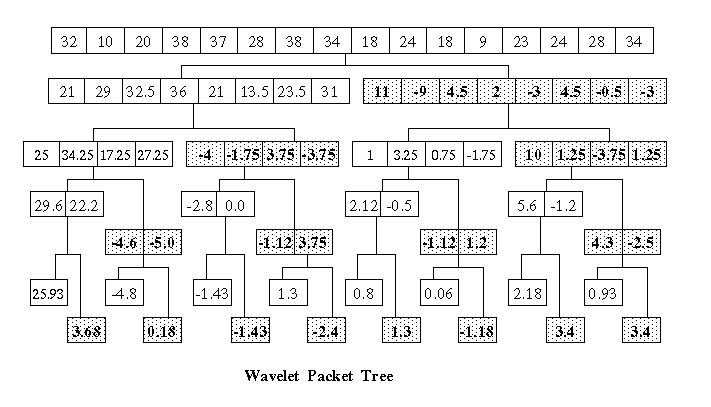The wavelet function used to construct the wavelet packet tree in Figure 1 is a version of the Haar wavelet that I refer to as the Haar classic wavelet function. Note that this differs from the lifting scheme version of the Haar wavelet.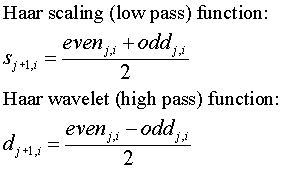The description of the wavelet packet transform on this web page and the implementation in the associated C++ code uses a tree form to represent the wavelet packet data. In the wavelet literature the result of the wavelet packet transform is sometimes referred to as a wavelet packet table.

### The Best Basis and Cost Functions

The wavelet basis is the range in the vector over which the scaling and wavelet functions are non-zero. For the Haar wavelet the basis is an increasing power of two, relative to the original data set. The first level following the data has a basis of two (the average and average difference is calculated on two elements). The next level has a basis of four, since the two elements used to calculate the scaling and wavelet functions are the result of four elements in the original data. The next level has a basis of eight, and so on.

The wavelet transform takes an input data set and represents it in a new form. No information is lost, however, and the result of the wavelet transform can be perfectly reconstructed into the original data. Assuming that the data is not random, the representation of the data produced by the wavelet transform may may be more compact (consisting of smaller values) than the original data set (see Predict Wavelets: wavelets viewed as compression).

From the point of view of compression, where we want as many small values as possible, the standard wavelet transform may not produce the best result, since it is limited to wavelet bases (the plural of basis) that increase by a power of two with each step. It could be that another combination of bases produce a more desirable representation.

The best basis algorithm finds a set of wavelet bases that provide the most desirable representation of the data relative to a particular cost function. A cost function may be chosen to fit a particular application. For example, in a compression algorithm the cost function might be the number of bits needed to represent the result. In Ripples in Mathematics the criteria for a cost function, K is given as

The value of the cost function is a real number. Given two vectors of finite length, a and b, we denote their concatenation by [a b]. This vector simply consists of the elements in a followed by the elements in b. We require the following two properties:

1. The cost function is additive in the sense that K([a b]) = K(a) + K(b) for all finite length vectors a and b.
2. K(0) = 0, where 0 denotes the zero vector

Section 8.2.2 Best Basis in Ripples in Mathematics

One of the simplest cost function is a threshold function, shown below (in a demented combination mathematical notation with C/C++):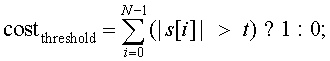The threshold cost function counts the number of values in a wavelet packet tree node whose absolute value is greater than a threshold value t. Figure 2 shows the threshold cost function values for the wavelet packet tree in Figure 1, where t = 1 (e.g., each number in figure 2 represents the number of values in that node whose absolute value is greater than 1).

Figure 2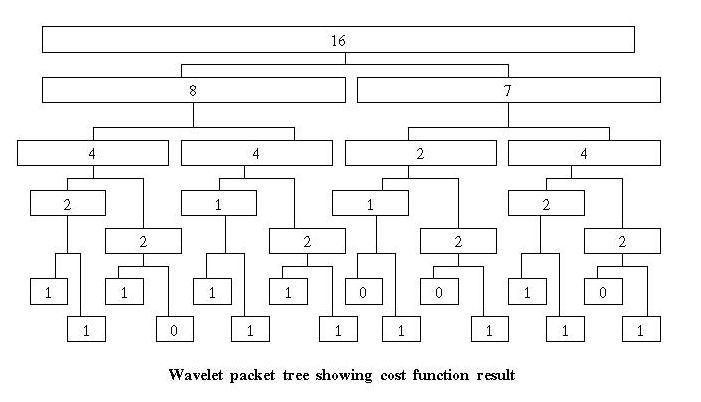To calculate the best basis, the tree is traversed and each node is marked with its cost value (relative to a particular cost function).

When the wavelet packet tree is constructed, all the leaves are marked with a flag. These "marks" are modified in calculating the best basis set. The best basis calculation is performed bottom up (that is, from the leaves of the tree toward the root):

• A leaf (a node at the bottom of the tree with no children) returns its cost value.

• As the calculation recurses up the tree toward the root, if there is a non-leaf node, v1 is the cost value for that node. The value v2 is the sum of the cost values of the children of the node.

• If (v1 <= v2) then we mark the node as part of the best basis set and remove any marks in the nodes in the sub-tree of the current node.

• If (v1 > v2) then the cost value of the node is replaced with v2.

The shaded nodes in Figure 3 show the nodes that are selected by the best basis algorithm given the costs calculated by the simple threshold function.

Figure 3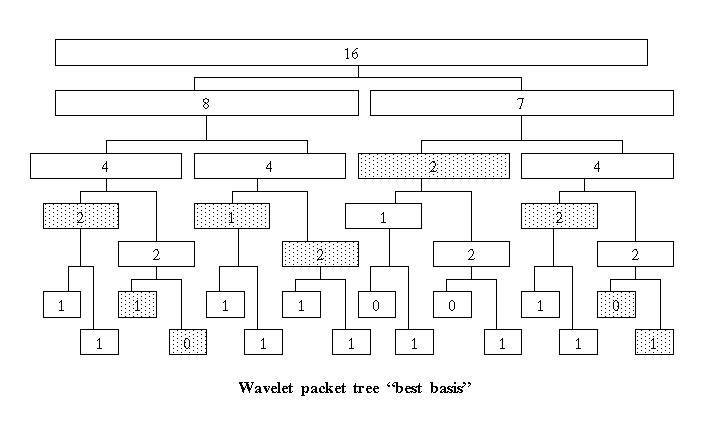The shaded values in Figure 4 show the wavelet packet transform values selected by the best basis algorithm. The best basis set can be obtained by traversing the tree, top down, from left to right. The traversal on a branch stops when a best basis node is encountered and this node is added to the best basis list.

Figure 4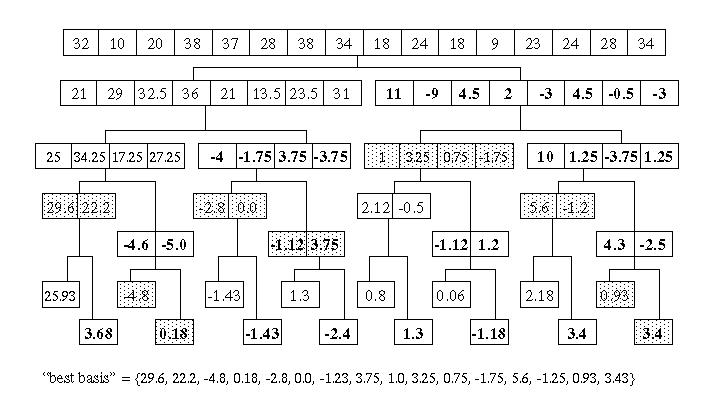The best basis set selected by the best basis algorithm is relative to a particular cost function. In some cases the best basis set may be the same set yielded by the wavelet transform (in which case we could have used the simpler algorithm). In other cases the best basis function may not yield a result that differs from the original data set (e.g., the original data set is already a minimal representation in terms of the cost function).

### Other Cost Functions

One cost function that has been proposed in the literature is the Shannon entropy function. A modified version of the Shannon entropy function is given in Ripples in Mathematics: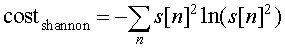The Shannon entropy function provides a measure of the economy of representation of a signal. It is usually applied to the probability of a character in a fixed alphabet. Ripples in Mathematics does not provide an explaination I could understand of how the standard version of the Shannon entropy function becomes the modified version.

The Shannon entropy cost function is included in the C++ code. Better results seem to be obtained when the result of the wavelet transform step is normalized (divided by the square root of two in the forward transform). An example can be found in haar.h Normalization is used to assure that the energy of the signal is constant at each step of the wavelet transform.

### The Inverse Transform from the Best Basis Set

In this example, the best basis data set consists of smaller values, so it is a more compact representation. If the wavelet packet transform and the best basis algorithm are used for data compression, there must be a way to recover the original data set from the best basis set. I have not seen any material on calculating the inverse transform from the best basis set, so this algorithm is entirely my own.

If the best basis set is represented as a list, where each element in the list is the node from the wavelet packet tree, we can recover the original data. A compression algorithm might represent such a list by an element count for each node, followed by the data elements. For such an scheme to be practical, enough compression would have to be yielded by the best basis algorithm to make up for the element count values that are inserted in the data set.

Logically, the inverse calculation must regenerate the wavelet packet tree from the best basis list. This is shown in Figure 5. The list is traversed from start to end. The first node, containing the values {29.6, 22.2} is read. A parent node, which is twice as large, is created and {29.6, 22.2} becomes the left child. This sub-tree is pushed on a stack. Next the node containing {-4.8} is read from the list. A tree created for this node (with a two element root) is smaller than the node on the top of the stack, so a new sub-tree is created and pushed on the stack. This node has two elements in the root and {-4.8} as the left child. The next value in the list is {0.18}. This value becomes the right hand side of the top of stack (TOS) sub tree. The inverse wavelet transform step is now calculated on the TOS sub-tree, yielding a two element result. This result now becomes the right hand size child of the next sub-tree in the stack and this sub-tree is reduced, yielding a four element node. This four element result become the left child of a new sub-tree, with an eight element root. The calculation proceeds as shown in Figure 5 B and C.

Figure 5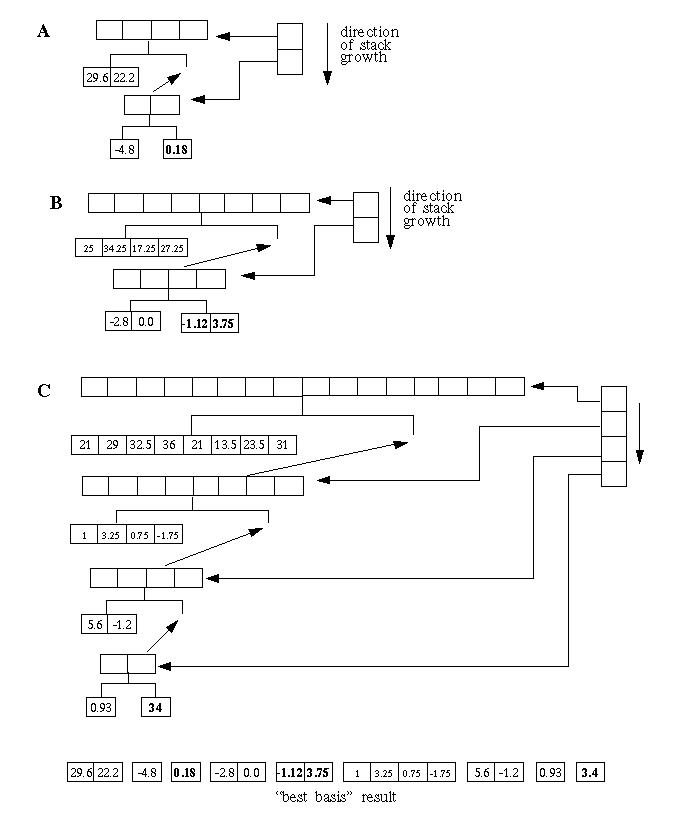The algorithm outlined in Figure 5 is implemented in the invpacktree class in the associated C++ code.

### Choosing C++ Over Java for the Wavelet Packet Transform

As long as the speed of execution is not a concern, Java provides a number of advantages. Java provides the same class abstraction as C++ in a smaller and easier to understand langauge. Because Java is a smaller language there is a better chance that published source code will compile and run the same way on different system. Java uses garbage collection, freeing the programmer from having to worry about memory recovery.

However, Java is currently missing two powerful language features: operator overloading and generics (in C++ generics are implemented via templates). Operator overloading allows containers to be created that reduce the amount of data copying when calculating the forward and inverse wavelet transform. Templates allow the wavelet transform algorithms to be expressed in generic form, allowing the wavelet transform to be applied to these containers.

### There is No Free Lunch (especially when it comes to C++)

C++ supports powerful language features that are missing from Java, but there is a price paid for this power. C++ is a much more complex language and it does not support garbage collection (although garbage collection packages are available for C++).

In implementing the wavelet packet transform and the best basis algorithm, I have tried to implement "professional grade" software. In C++ this means that memory must be recovered properly, since garbage collection cannot be relied upon as it can in Java. In C++ objects are allocated using the new operator. They can be recovered usign the delete operator. There are a number of problems with using delete:

1. The delete operator incurs overhead (i.e., it is slow)
2. Recovering memory using delete leads to more complicated code
3. Using delete can lead to errors and memory leaks

One way around using delete is to use a memory pool. This approach is followed in the wavelet packet transform code. Objects are allocated from a memory pool. The entire pool can be recovered when the objects are no longer needed. This allows new to be used without worrying about calling delete. Use of a memory pool requires overloading the new operator (see Overloading New in C++).

### C++ versus MATLAB

The C++ source code (including comments and white space) consists of almost 4,000 lines. This is considerably larger than the MATLAB code published in Ripples in Mathematics for the wavelet packet transform and the best basis algorithm. The C++ code supports more functions however, including display of the wavelet packet tree and best basis set. The core algorithms seem about the same size.

However, the trade off between MATLAB and C++ is moot, at least for me. MATLAB looks like a great product. It is probably the most popular computer mathematics package. MATLAB has a wide variety of "toolboxes", including toolboxes for statistics and wavelets. You can even get packages that support SQL database references and connect your MATLAB code to data feeds like Reuters stock market data. Very cool! However, I can't afford MATLAB. At the time I wrote this web page the base cost of MATLAB was \$1,900 US. The statistics package costs \$600 and the wavelet package costs \$900. So don't send me e-mail telling me how much better MATLAB is unless you can get me a free (and legal) copy (and, while you're at it, I'd really like the statistics package too).

The C++ source code for the wavelet packet algorithms can be downloaded here in tar format or here in zip format.

This source code includes the forward and inverse wavelet packet algorithms, "best basis" calculation and the frequency ordered wavelet packet tree creation for wavelet time/frequency analysis.

Test programs are provided for the wavelet packet and frequency ordered wavelet packet algorithms.

I have included Makefiles for Windows nmake and UNIX GNU make.

I find Windows project files spectacularly unportable, so I try never to use them when publishing code. If you don't know how to use make (or nmake), I recommend referring to either the Windows developer documentation or the appropriate O'Reilly book.

#### tar

The software published on these pages is packaged in "tar" format. The tar program is a UNIX archiving program. Perhaps because I'm a long time UNIX hacker, I prefer tar to the zip utilities, at least for small amounts of data where compression is not a concern (and then I prefer gzip, the GNU compression program). The tar program can be found on all UNIX systems, Linux and, I assume, Apple's OS X. You can down load tar for Windows NT (Win32) here. This version of tar is from Cygnus (now Red Hat) and is compiled from FSF Copy Left source.

### View the Doxygen Generated Documentation

I have documented the wavelet packet source code using doxygen formatted comments. These comments are meant to be read in combination with this web page. The doxygen generated documentation can be found here

### References

• Ripples in Mathematics: The Discrete Wavelet Transform by Jensen and la Cour-Harbo, Springer Verlag, 2001
• Wavelet Methods for Time Series Analysis by Percival and Walden, Cambridge University Press, 2000

Ian Kaplan
March 2002
Revised: